## Introduction

Studies of phase transitions and critical behaviour in non-Hermitian systems date back to the discovery of the Lee-Yang edge singularity1, where an imaginary magnetic field in the high-temperature Ising model was demonstrated to trigger an exotic phase transition. More recently, the real-to-complex spectral phase transition has been found in a broad class of non-Hermitian Hamiltonians that satisfy parity-time (PT) symmetry2. While such systems were once considered to be of purely academic interest, related questions are now within experimental reach3,4,5,6,7.

A Hamiltonian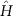is said to be PT-symmetric if it commutes with the combined operator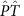, but not necessarily with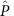andseparately. Hereand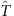are the parity and time-reversal operators, respectively. PT symmetry is said to be unbroken if every eigenstate ofis PT-symmetric; then, the entire spectrum is real even thoughis not Hermitian. PT symmetry is said to be spontaneously broken if some eigenstates ofare not the eigenstates of the PT operator even though; then, some pairs of eigenvalues ofbecome complex conjugate to each other. PT symmetry breaking is typically accompanied by the coalescence of eigenstates and that of the corresponding eigenvalues at an exceptional point8 in the discrete spectrum or the spectral singularity9 in the continuum spectrum. While these features also hold for a certain class of antilinear symmetries10, PT symmetry allows experimental implementations by spatial engineering of gain–loss structures, leading to a rich interplay between theory and experiment in optics4,5,6,7,11, superconductors12, atomic physics13 and optomechanics14. In particular, the real-to-complex spectral transition (PT transition) has been observed in experiments of classical systems15. In all these developments, however, many-body correlations still play no role.

Quantum critical phenomena, in contrast, arise from collective behaviour of strongly correlated systems and exhibit universal long-distance properties. In view of recent developments in designing open many-body systems in ultracold atoms16,17,18,19,20 and exciton–polariton condensates21, it seems ripe to explore the role of PT symmetry in quantum critical phenomena and ask whether or not the concept of the universality need be extended in synthetic non-conservative systems.

Here we report that a combination of spectral singularity and quantum criticality yields an exotic critical point in the extended parameter space and that, in the PT-broken phase, a local gain–loss structure results in an anomalous enhancement of superfluid correlation owing to semicircular renormalization group (RG) flows. This contrasts sharply with the suppression of superfluid correlation due to hyperbolic RG flows in the Berezinskii–Kosterlitz–Thouless (BKT) paradigm. Our findings demonstrate that the interplay between many-body correlations and PT symmetry leads to the emergence of quantum critical phenomena beyond the Hermitian paradigm of quantum many-body physics.

## Results

### Parity-time-symmetric sine-Gordon model

We consider a class of one-dimensional (1D) quantum systems described by the field theory Hamiltonianwhereis a scalar field,is its conjugate momentum satisfying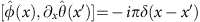, andis a potential for the field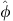. Without the potential term, equation (1) is known as the Tomonaga-Luttinger liquid (TLL) Hamiltonian, which gives a universal framework for describing 1D interacting bosons and fermions22. Here, v is the sound velocity, the TLL parameter K characterizes the interaction strength, and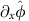andare related to the density and the Josephson phase, respectively. The introduction of the cosine potentialresults in the sine-Gordon model, which describes the BKT transition to a gapped phase. For bosons on a lattice, this corresponds to a superfluid-to-Mott-insulator (MI) transition23. Here we consider a generalization to the PT-symmetric case by adding an imaginary contribution to the potential term as follows:where αr and αi characterize the strengths of the real and imaginary parts of the potential. When the real part becomes relevant, it suppresses the fluctuations of, stabilizing a non-critical, gapped phase. In contrast, if the imaginary part is relevant, it facilitates the fluctuations ofand enhances correlation in the conjugate field, as we will see later. The field theory (1) with the potential (2) satisfies PT symmetry since the fieldhas odd parity. The PT-symmetric Hamiltoniancan be implemented by a continuously monitored 1D interacting ultracold atoms (see Supplementary Note 1 and Supplementary Fig. 1).

We note that if αr>αi,has a real spectrum and thus PT symmetry is unbroken. This can be proved by the theorem24 which states that the spectrum is real if and only if there exists an operator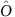satisfying, whereis a Hermitian operator. Indeed, we can explicitly construct such an operator for αr>αi by the choice of, whereis a constant part ofand η ≡ arctanh(αi/αr). Then, the potential term in the effective field theory is transformed toandreduces to the sine-Gordon Hamiltonian25. Divergence of η at αr=αi signals spontaneous breaking of PT symmetry.

### Renormalization group analysis

To unravel the universal critical behaviour of the PT-symmetric Hamiltonian, we perform an RG analysis26 to obtain the following set of flow equations which are valid up to the third order in gr,i:Here l is the logarithmic RG scale and gr,i ≡ αr,ia2/(ħv) are the dimensionless coupling constants with a being a short-distance cut-off. The velocity v stays constant to all orders in gr,i because of the Lorentz invariance of the theory. In contrast to the two-dimensional phase diagram of the conventional sine-Gordon model, the PT-symmetric system has the three-dimensional (3D) phase diagram (Fig. 1a). When PT symmetry is unbroken, that is, gi<gr, the spectrum is equivalent to that of the closed system as discussed above and the conventional RG flow diagram with hyperbolic flows is reproduced (Fig. 1b). Here the BKT boundary between the superfluid TLL phase and the MI phase extends over the curved surface. We note that the operatordoes not affect the critical properties of the ground state since it only modifies the zero modes associated with the field. Since the non-Hermitian term can arise from the measurement backaction, the quantum phase transition induced by increasing gi may be regarded as measurement-induced.

In the strongly correlated regime K<2, a new type of quantum phase transition appears on the PT threshold plane gi=gr. This phase transition is accompanied by spontaneous breaking of PT symmetry in eigenstates, contrary to the ordinary BKT transition exhibiting no symmetry breaking. The BKT and PT phase boundaries merge on the line defined by K=2 and gi=gr (Fig. 1c). In general, at the PT symmetry-breaking point, the spectral singularity9 arises where two or more eigenvalues as well as their eigenstates coalesce in the continuum spectrum. In optics, the spectral singularity leads to unidirectional wave phenomena5. In contrast, in many-body systems, the coexistence of the spectral singularity and the quantum criticality at gi=gr and K=2 results in what we term a spectral singular critical point, which represents a unique universality class in non-conservative systems.

When the PT symmetry is broken, that is, gi>gr, unconventional RG flows emerge: starting from the K<2 side, gr,i and K initially increase, and after entering the K>2 side, the flow winds and converges to the fixed line with gr,i=0 (Fig. 1d). Physically, this significant increase in the TLL parameter K indicates that the superfluid correlation decays more slowly and is thus enhanced by the non-Hermiticity of an imaginary potential. The enhancement is viewed as anomalous because, in the conventional BKT paradigm, a real potential suppresses the fluctuation ofand stabilizes the gapped MI phase for K<2. Moreover, owing to the semicircular RG flows, the imaginary potential allows for a substantial increase of the TLL parameter K even if its strength gi is initially very small. The PT-broken phase exhibits other observable consequences such as anomalous lasing and absorption as observed in optics27 (see Supplementary Note 2 for the experimental implementation in ultracold atoms).

### Ground-state phase diagram of the lattice model

To numerically demonstrate these findings, we introduce a lattice Hamiltonianwhose low-energy behaviour is described by the PT-symmetric effective field theory. Hereare the spin–1/2 operators at site m and the parameters (−Δ, hs, γ) are related to (K, gr, gi) in the field theory, where we set J=1. The non-Hermitian term represents a periodic gain–loss structure and effectively strengthens the amplitude of the hopping term, leading to enhanced superfluid correlation. The determined phase diagram and a typical exact finite-size spectrum are shown in Fig. 2. The BKT transition is identified as a crossing point of appropriate energy levels28 and the PT threshold is determined as a coalescence point in low-energy levels, as detailed in Methods section and Supplementary Methods. The coalescence point is found to be an exceptional point from the characteristic square-root scaling8 of the energy gap (see the inset figure in Fig. 2b). We note that, above the PT threshold, some highly excited states turn out to have positive imaginary parts of eigenvalues and cause the instability in the long-time limit. The presence of such high-energy unstable modes is reminiscent of parametric instabilities in exciton–polariton systems29, and can ultimately destroy the 1D coherence30. In our set-up, where the imaginary term is adiabatically ramped up, the amplitudes of these unstable modes can greatly be suppressed and the system can remain, with almost unit fidelity, in the ground state in which the critical behaviour is sustained (see Supplementary Note 3 and Supplementary Figs 2 and 3 for details).

### Numerical demonstration of enhanced superfluid correlation

To demonstrate the anomalous enhancement of superfluid correlation in the PT-broken regime, we have performed numerical simulations using the infinite time-evolving block decimation (iTEBD) algorithm31. The correlation function exhibits the critical decay with a varying critical exponent and the corresponding TLL parameter significantly increases, surpassing K=2 as shown in Fig. 3. Physically, this enhancement of superfluid correlation at long distances can be interpreted as follows. A local gain–loss structure introduced by the imaginary term causes locally equilibrated flows15 in the ground state. This results in the enhancement of fluctuations in the density, or equivalently, the suppression of fluctuations in the conjugate phase. It is this effect that increases the superfluid correlation. The numerical results are consistent with the analytical arguments given above, and demonstrate that the RG analysis is instrumental in studying critical properties of a non-Hermitian many-body system.Figure 3: Anomalous enhancement of superfluid correlation in the PT-broken quantum critical phase.

### Experimental realization in a one-dimensional Bose gas

The PT-symmetric many-body Hamiltoniandiscussed above can be implemented in a 1D interacting ultracold bosonic atoms subject to a shallow PT-symmetric optical lattice V(x)=Vr cos(2πx/d)−iVi sin(2πx/d), where Vr and Vi are the depths of the real and imaginary parts of a complex potential and d is the lattice constant. An imaginary optical potential can be realized by using a weak near-resonant standing-wave light (Methods section). Since V(x) remains invariant under simultaneous parity operation (x→−x) and time reversal (that is, complex conjugation), the system satisfies the condition of PT symmetry (Fig. 4a). In open quantum systems, by postselecting null measurement outcomes, the time evolution is governed by an effective non-Hermitian Hamiltonian32,33,34. The achieved experimental fidelity has already been high enough to allow experimenters to implement various types of postselections35,36,37. The low-energy behaviour of this system is then described by the PT-symmetric effective field theory. We note that the lattice Hamiltonian (4) can also be realized in ultracold atoms by superimposing a deep lattice that does not influence the universal critical behaviour (Fig. 4b).Figure 4: Experimental set-up of a PT-symmetric many-body system in ultracold atoms.

We stress that the dynamics considered here is different from the one described by a master equation, where dissipative processes, in general, tend to destroy correlations underlying quantum critical phenomena. In contrast, the postselections allow us to study the system free from the dissipative jump processes, while non-trivial effects due to measurement backaction still occur via the non-Hermitian contributions in the effective Hamiltonian.

## Discussion

The reported fixed points in the extended parameter space suggest that an interplay between spectral singularity and quantum criticality results in an exotic universality class beyond the conventional paradigm. It remains an open question how the universality accompanying spectral singularity found in this work is related to non-unitary conformal field theories (CFT) studied in various fields ranging from statistical mechanics38 to high-energy physics39. It is particularly notable that a certain critical point of the integrable spin chain with PT-symmetric boundary fields corresponds to an exceptional point and is believed to be described by non-unitary CFT40. This suggests an intimate connection between the spectral singular critical point and the non-unitary CFT. Given recent success in measuring entanglement entropy in ultracold atoms37, it is of interest to study how quantum entanglement behaves in the presence of spectral singularity. In the PT-broken phase, we have shown that the ground state exhibits the enhanced superfluid correlation indicating the tighter binding of the topological excitations, in stark contrast to their proliferation as found in the BKT paradigm. In Hermitian systems, a relevant perturbation around RG fixed points has a tendency to suppress fluctuations of the concerned field and stabilize a non-critical, gapped phase. Our finding indicates that a relevant imaginary perturbation can realize the opposite situation of enhancing fluctuations of the concerned field and facilitating correlation in the conjugate field. An exploration of such unconventional quantum criticality in other synthetic, non-conservative many-body systems presents an interesting challenge. Further studies in these directions, together with their possible experimental realizations, could widen applications to future quantum metamaterials.

## Methods

### Details of numerical calculations

The phase diagram in Fig. 2a is determined from the exact diagonalization analysis of the lattice Hamiltonian (4). To identify the BKT transition point, we calculate the exact finite-size spectrum and find a crossing of low-energy levels having appropriate quantum numbers28. The PT transition point is identified as the first coalescence point in the low-energy spectrum with increasing γ. The calculations are done for different system sizes and the final results are obtained through extrapolation of the data to the thermodynamic limit. Further details are given in Supplementary Methods and Supplementary Fig. 4. The correlation function and the associated variation of the TLL parameter K shown in Fig. 3 are calculated by applying the iTEBD algorithm31. We emphasize that this method can be applied to study the ground-state properties of the non-Hermitian system. The method can accurately calculate the imaginary-time evolutionfor an infinite system size, where τ is an imaginary time,is an initial state anddenotes the norm of the state. In the limit of large τ, we obtain the quantum state, the real part of which eigenvalue is the lowest in the entire spectrum, that is, an effective ground state of a non-Hermitian system. We note that the imaginary part of the eigenvalue does not affect the calculation since it only changes an overall phase of the wavefunction in the imaginary-time evolution. We then determine the TLL parameter K from the calculated correlation function by using the relation.

### Derivation of the low-energy field theory of ultracold atoms

Here we explain the derivation of the low-energy effective field theory (1) of ultracold atoms. We start from the Hamiltonian in which the periodic potential Vr cos(2πx/d) is added to the Lieb–Liniger model41. Then, we introduce an imaginary optical lattice potential by using a weak near-resonant standing-wave light. This scheme is possible if the excited stateof an atom has decay modes other than the initial ground stateand its decay rate is faster than the spontaneous decay rate fromtoand the Rabi frequency42,43,44 (Fig. 4a). Such a condition can be satisfied by, for example, using appropriate atomic levels45 or light-induced transitions16. The difference between the wavelengths of the real and imaginary periodic potentials caused by different detunings of the lasers can be negligible. Using a second-order perturbation theory8 for the Rabi coupling and adiabatically eliminating the excited state, we obtain an effective time-evolution equation for the ground-state atoms. We then assume that null measurement outcomes are postselected so that the dynamics is described by the non-Hermitian Hamiltonian32,33,34. In this situation, the overall imaginary constant in the eigenvalue spectrum does not affect the dynamics since it can be eliminated when we normalize the quantum state, leading to the imaginary potential iVi sin(2πx/d). Finally, we follow the standard procedure22 of taking the low-energy limit of the model and arrive at the Hamiltonian (1). The details of the calculations and experimental accessibility in ultracold atoms are described in Supplementary Notes 1 and 2.

### Data availability

The data that support the plots within this paper and other findings of this study are available from the corresponding author on request.